# 基于ICL7107构建的数字直流电压表电路

#### 作者芯片半导体

6月 30, 2022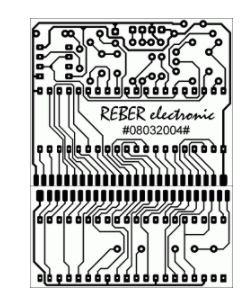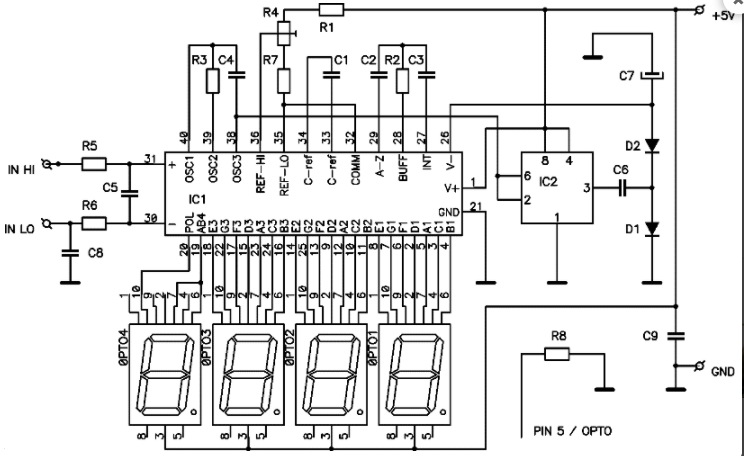R1 = 8K2

R2 = 47K / 470K

R3 = 100K

R4 = 2K

R5， R6 = 47K

R7 = 0R / 4K7

R8 = 560R

C1，C5， C6， C8， C9 = 100n

C2 = 470n / 47n

C3 = 220n

C4 = 100p

C7 = 10-22u

D1， D2 = 1N4148

IC1 = ICL7107

IC2 = NE555

OPTO = CA 10针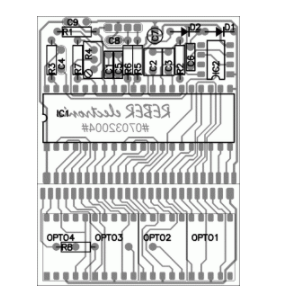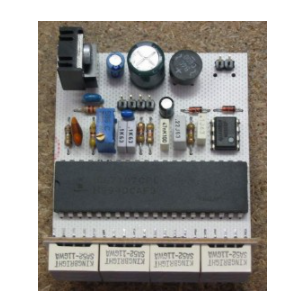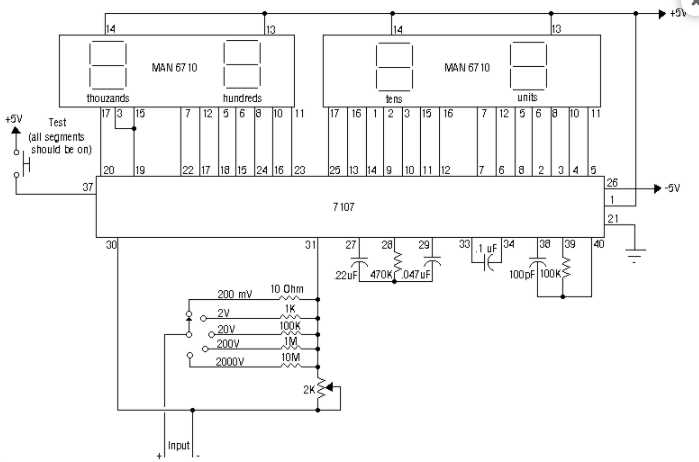• ICL7107